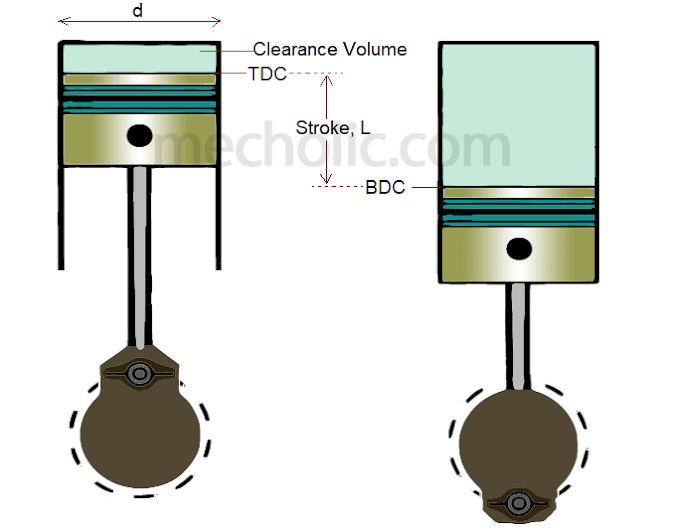Nomenclature or Terminology Used In Study of IC Engine | Mecholic -->

# Nomenclature or Terminology Used In Study of IC EngineThe common terms used in calculations and study of IC engine is listed below.

Cylinder Bore (d): Cylinder bore is the inner diameter of working cylinder. It is represented in millimetre (mm).

Piston Area (A): Piston area is defined as the area of a circle of diameter equal to the cylinder bore. It is usually represented in square centimetre (cm2).
A= (π/4) d2

Dead centres: it is the position of piston and moving parts connected to it, at the moment piston reverse its direction of motion. This happen either end of the stroke. There are two dead centre.

Top dead centre (TDC): When the piston farthest from the crankshaft (for vertical engine). In a horizontal engine, it is called inner dead canter (IDC).

Bottom dead centre (BDC): When the piston is nearest to the crankshaft. In a horizontal engine, it is equivalent to outer dead centre (ODC).

Stroke (L): Stroke is the maximum distance through which the piston moves while engine running (distance covered between two successive reversal of motion of piston) or distance travelled by the piston from BDC to TDC.  It is usually represented in millimetre (mm).

Stroke to bore ratio: It is the ratio of the stroke of engine (L) to its cylinder bore (d).
Stroke to bore ratio = L/d
Stroke to bore ratio is an important parameter in the classification of engine size.
If L/d = 1, or d=L then engine called square engine.
If L/d > 1, or d<L then engine called under-square engine.
If L/d < 1, or d>L then engine called over-square engine.
Over-square engines have shorter stroke length and it allows to engine operates at high speed.

Displacement volume or Swept volume (Vs): it is the volume swept by the piston when moving from one dead centre to the other dead centre. Swept volume usually expressed in cubic centimetre (cc).
Vs = A × L = (π/4) d2 L

Clearance volume (Vc): it is the volume of the combustion chamber above the piston when it at top dead centre.

Cubic capacity or Engine capacity: Engine capacity = Swept volume × Number of cylinder
Engine capacity = Vs × K

Compression ratio (γ): it is the ratio of total volume of the cylinder when piston at BDC to the clearance volume.
γ = VT/Vc =(Vs+ Vc)/Vc = 1+ (Vs/Vc)
Compression ratio is an important parameter to define the type and efficiency of the engine.